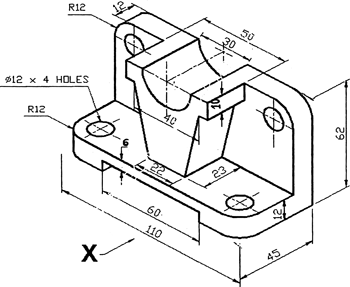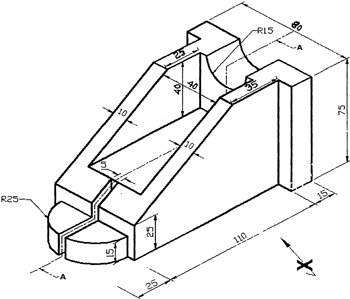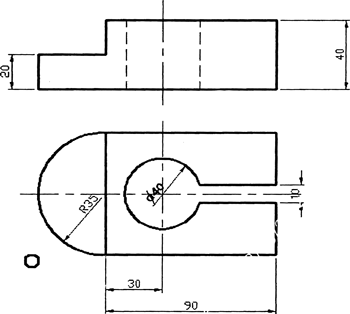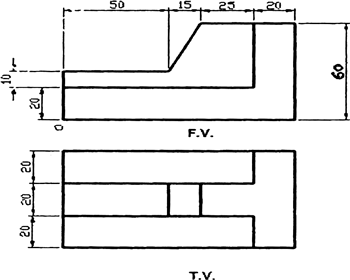MORE IN Engineering Drawing
MU First Year Engineering (Semester 2)
Engineering Drawing
December 2014
Total marks: --
Total time: --
INSTRUCTIONS
(1) Assume appropriate data and state your reasons
(2) Marks are given to the right of every question
(3) Draw neat diagrams wherever necessary

1(a) Draw involute of a circle of 60 mm diameter. Also draw the normal and tangent at a point on the curve at a distance of 120 mm from the centre of the circle.
6 M
1(b) The pictorial view of a machine part is given in the figure. Draw the following views:
1. Front View
2. Top View9 M

2 Figure given below shows the pictorial view of an object. Draw to full scale the following views:
1. Sectional front view (section AA)
2. Top view
3. Left hand side view
4. Insert 10 major dimensions15 M

3 A square pyramid of side of base 40 mm axis 70 mm has one of the triangular faces on the VP. Draw the projections when the front view of the axis is inclined 30° to the HP, keeping the apex nearer to the observer.
15 M

4 A right circular cone of base circle diameter 50 mm and axis 60 mm long is resting on its base on HP. It is cut by a section plane which is perpendicular to VP and inclined to HP such that the plane is parallel to the end generator and 10 mm away from it. Draw the front view, the sectional top view and the true shape of section. Also draw the development of the cone after removing the portion containing the apex.
15 M

5(a) A hexagonal prism of 35 mm edge of base and 70 mm length of axis is having an edge of base in the HP and the rectangular face containing that edge is inclined 30° to HP and perpendicular to VP. Draw the projections.
6 M
5(b) The orthographic projection of an object is given in the figure below. Draw the isometric view:9 M

6(a) The distance between the end projectors of a line AB is 40 mm. The end point A is 15 mm above the HP and 20 mm in front of the VP. The line is inclined 30° to the HP. Draw the projections if the true length of the line is 80 mm. Find its inclinaton with the VP. Take end point B in the 1st quadrant.
7 M
6(b) Draw the isometric view of the given views:8 M

More question papers from Engineering Drawing